Subsections

# 2.3ArrayQueue: An Array-Based Queue

In this section, we present the ArrayQueue data structure, which implements a FIFO (first-in-first-out) queue; elements are removed (using the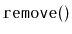operation) from the queue in the same order they are added (using the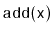operation).

Notice that an ArrayStack is a poor choice for an implementation of a FIFO queue. The reason is that we must choose one end of the list to add to and then remove from the other end. One of the two operations must work on the head of the list, which involves calling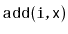or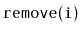with a value of. This gives a running time proportional to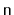.

To obtain an efficient array-based implementation of a queue, we first notice that the problem would be easy if we had an infinite array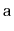. We could maintain one index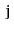that keeps track of the next element to remove and an integerthat counts the number of elements in the queue. The queue elements would always be stored in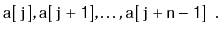Initially, bothandwould be set to 0. To add an element, we would place it in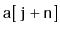and increment. To remove an element, we would remove it from, increment, and decrement.

Of course, the problem with this solution is that it requires an infinite array. An ArrayQueue simulates this by using a finite arrayand modular arithmetic. This is the kind of arithmetic used when we are talking about the time of day. For example 10 o'clock plus 5 hours gives 3 o'clock. Formally, we say that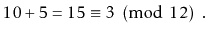We read the latter part of this equation as 15 is congruent to 3 modulo 12.'' We can also treat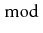as a binary operator, so that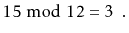More generally, for an integer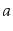and positive integer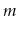,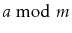is the unique integer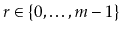such that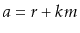for some integer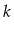. Less formally, the valueis the remainder we get when we divideby. In many programming languages, including Java, theoperator is represented using the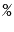symbol.2.2

Modular arithmetic is useful for simulating an infinite array, since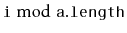always gives a value in the range. Using modular arithmetic we can store the queue elements at array locations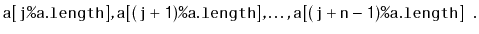This treatslike a circular array in which array indices exceeding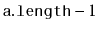wrap around'' to the beginning of the array.

The only remaining thing to worry about is taking care that the number of elements in the ArrayQueue does not exceed the size of.

    T[] a;
int j;
int n;


A sequence ofandoperations on an ArrayQueue is illustrated in Figure 2.2. To implement, we first check ifis full and, if necessary, call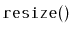to increase the size of. Next, we store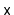in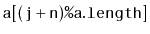and increment.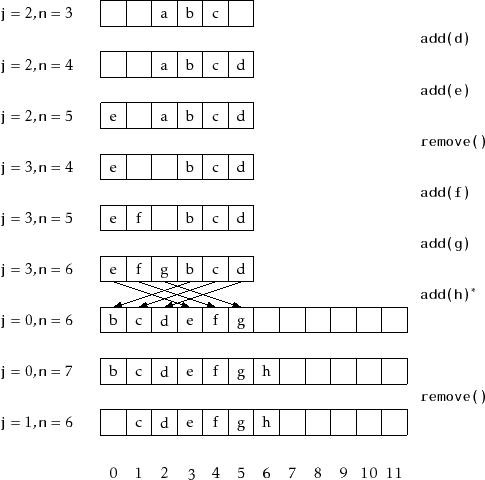boolean add(T x) {
if (n + 1 > a.length) resize();
a[(j+n) % a.length] = x;
n++;
return true;
}


To implementwe first storeso that we can return it later. Next, we decrementand increment(modulo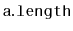) by setting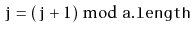. Finally, we return the stored value of. If necessary, we may callto decrease the size of.

    T remove() {
if (n == 0) throw new NoSuchElementException();
T x = a[j];
j = (j + 1) % a.length;
n--;
if (a.length >= 3*n) resize();
return x;
}


Finally, theoperation is very similar to theoperation of ArrayStack. It allocates a new arrayof size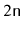and copies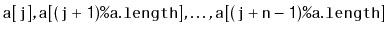onto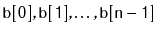and sets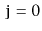.

    void resize() {
T[] b = newArray(Math.max(1,n*2));
for (int k = 0; k < n; k++)
b[k] = a[(j+k) % a.length];
a = b;
j = 0;
}


## 2.3.1 Summary

The following theorem summarizes the performance of the ArrayQueue data structure:

Theorem 2..2   An ArrayQueue implements the (FIFO) Queue interface. Ignoring the cost of calls to, an ArrayQueue supports the operationsandin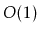time per operation. Furthermore, beginning with an empty ArrayQueue, any sequence ofandoperations results in a total of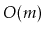time spent during all calls to.

#### Footnotes

... symbol.2.2
This is sometimes referred to as the brain-dead mod operator since it does not correctly implement the mathematical mod operator when the first argument is negative.
opendatastructures.org